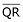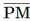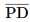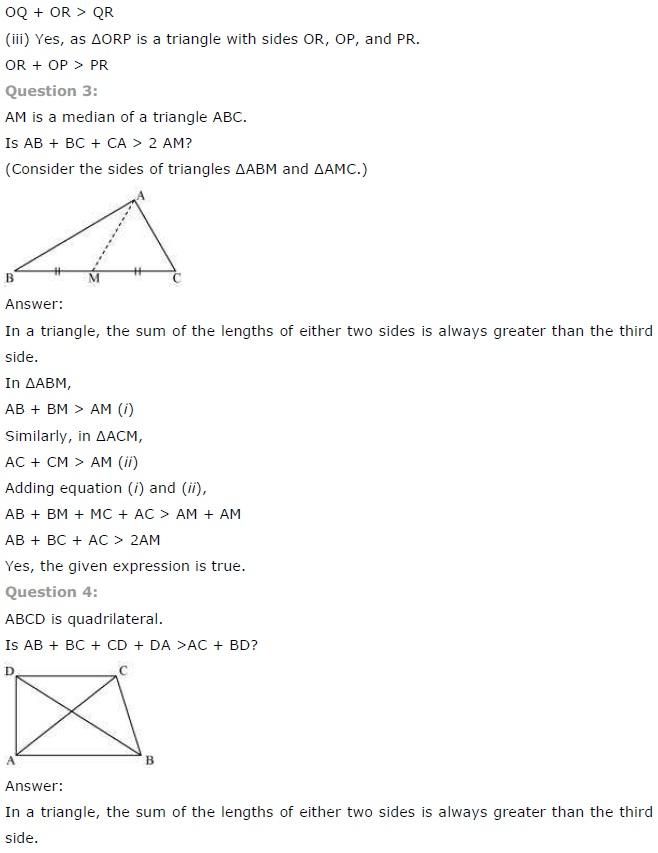# class 7 Maths Chapter-6 Triangle and its properties

### Exercise-6.1

Question 1:

In ∆PQR ,D is the mid-point of .

is __________.

PD is ___________.

Is QM = MR?

In the above given question, we have

∆PQR

Also, given that:

D is the mid-point ofTherefore,is altitude

Also,is Median

And,

QM ≠ MR

Question 2:

Draw rough sketches for the following:

(a) In ∆ABC, BE is a median.

(b) In  ∆PQR ,PQ and PR are altitudes of the triangle.

(c) In ∆XYZ, YL is an altitude in the exterior of the triangle.

(a) In ∆ABC, BE is a median.

We have,

∆ABC,

And in this triangle BE is a median

Therefore,

The rough sketch for the given figure is as follows:

(b) In  ∆PQR ,PQ and PR are altitudes of the triangle.

We have,

∆PQR,

And in this triangle PQ and QR are the altitudes of the triangle

Therefore,

The rough sketch for the given figure is as follows:

(c) In ∆XYZ, YL is an altitude in the exterior of the triangle.

We have,

∆XYZ,

And in this triangle YL is an altitude in the exterior of the triangle

Therefore,

The rough sketch for the given figure is as follows:

Question 3:

Verify by drawing a diagram, if the median and altitude of an isosceles triangle can be same.

We have to prove that:

The median and altitude of an isosceles triangle are same

The diagram for the given question is as follows:

This can be proved as follows:

First of all we have to draw a line segment AD which is perpendicular to BC. This is an altitude for this triangle

Now,

From the figure it can also be observed that the length of BD and DC is also same

Therefore,

AD is also median of this isosceles triangle

Hence, proved

### Exercise-6.2

Question 1:

Find the value of the unknown exterior angles x in the following diagrams:

(i) It is given in the question that,

1st interior angle = 50o and 2nd interior angle = 70o

Note: According to exterior angle theorem,

The measure of an exterior angle of a triangle is equal to the sum of the measure of the two non-adjacent interior angles of the triangle.

Exterior angle = x

Sum of interior angles = 50o + 70o

Thus, using exterior angle theorem,

x = 50o + 70o

x = 120o

∴ The value of x is 120o

(ii) It is given in the question that,

1st interior angle = 65o and 2nd interior angle = 45o

Note: According to exterior angle theorem,

The measure of an exterior angle of a triangle is equal to the sum of the measure of the two non-adjacent interior angles of the triangle.

Exterior angle = x

Sum of interior angles = 65o + 45o

Using, exterior angle theorem, we have

x = 65o + 45o

x = 110o

Hence, the value of x is 110o

(iii) It is given in the question that,

1st interior angle = 30o and 2nd interior angle = 40o

Note: According to exterior angle theorem,

The measure of an exterior angle of a triangle is equal to the sum of the measure of the two non-adjacent interior angles of the triangle.

Exterior angle = x

Sum of interior angles = 30o + 40o

Using exterior angle theorem, we have

x = 30o + 40o

x = 70o

Hence, the value of x is 70o

(iv) It is given in the question that,

1st interior angle = 60o and 2nd interior angle = 60o

Note: According to exterior angle theorem,

The measure of an exterior angle of a triangle is equal to the sum of the measure of the two non-adjacent interior angles of the triangle.

Exterior angle = x

Sum of interior angles = 60o + 60o

Using exterior angle theorem, we have

x = 60o + 60o

x = 120o

Hence, the value of x is 120o

(v) It is given in the question that,

1st interior angle = 50o and 2nd interior angle = 50o

Note: According to exterior angle theorem:

The measure of an exterior angle of a triangle is equal to the sum of the measure of the two non-adjacent interior angles of the triangle.

Exterior angle = x

Sum of interior angles = 50o + 50o

Using exterior angle theorem, we have

x = 50o + 50o

x = 100o

Hence, the value of x is 100o

(vi) It is given in the question that,

1st interior angle = 30o and 2nd interior angle = 60o

Note: According to exterior angle theorem:

The measure of an exterior angle of a triangle is equal to the sum of the measure of the two non-adjacent interior angles of the triangle.

Exterior angle = x

Sum of interior angles = 30o + 60o

Using, exterior angle theorem, we have

x = 30o + 60o

x = 90o

Hence, the value of x is 120o

Question 2:

Find the value of the unknown interior angle x in the following figures:

### Exercise-6.3

Question 1:

Find the value of the unknown x in the following diagrams:

(i) In the above question, we have

1st interior angle = x, 2nd interior angle = 50o and 3rd interior angle = 60o

We have to find out the value of x

Thus,

x + 50o + 60o = 180o  (angle sum property of triangle)

x + 110o = 180o

x = 180o – 110o

x = 70o

Hence, the value of x is 70o

(ii) In the above question, we have

1st interior angle = x, 2nd interior angle = 90o and 3rd interior angle = 30o

We have to find out the value of x

Thus,

x + 90o + 30o = 180o   (angle sum property of triangle)

x + 120o = 180o

x = 180o – 120o

x = 60o

Hence, the value of x is 60o

(iii) In the above question, we have

1st interior angle = x, 2nd interior angle = 30o and 3rd interior angle = 110o

We have to find out the value of x

Thus,

x + 30o + 110o = 180o (angle sum property of triangle)

x + 140o = 180o

x = 180o – 140o

x = 40o

Hence, the value of x is 40o

(iv) In the above question, we have

1st interior angle = 50o, 2nd interior angle = x and 3rd interior angle = x

We have to find out the value of x

Thus,

50o + x + x = 180o    (angle sum property of triangle)

2x + 50o = 180o

2x = 180o – 50o

2x = 130o

x = 130/2

x = 65o

Hence, the value of x is 65o

(v) In the above question, we have

1st interior angle = x, 2nd interior angle = x and 3rd interior angle = x

We have to find out the value of x

And,

x + x + x = 180o     (angle sum property of triangle)

3x = 180o

### Exercise-6.4

Question 1:
Is it possible to have a triangle with the following sides?
(i) 2 cm, 3 cm, 5 cm
(ii) 3 cm, 6 cm, 7 cm
(iii) 6 cm, 3 cm, 2 cm

(i) 2 cm, 3 cm, 5 cm
We know that,
In a triangle, the sum of the length of either two sides of the triangle is always greater than the third side
The given sides of triangle in this question are:
2 cm, 3 cm and 5 cm
Now, 2 + 3 + = 5 cm
5 cm = 5 cm
Hence,
The triangle is not possible as the sum of the length of either two sides of the triangle is not greater than the third side

(ii) 3 cm, 6 cm, 7 cm
We know that,
In a triangle, the sum of the length of either two sides of the triangle is always greater than the third side
The given sides of triangle in this question are:
3 cm, 6 cm and 7 cm
Now, 3 + 6 = 9 cm and 9 cm > 7 cm
6 + 7 = 13 cm and, 13 cm > 3 cm
3 + 7 = 10 cm and, 10 cm > 6 cm
Hence,
The triangle is possible as the sum of the length of either two sides of the triangle is greater than the third side

(iii) 6 cm, 3 cm, 2 cm
We know that,
In a triangle,
The sum of the length of either two sides of the triangle is always greater than the third side
Therefore,
The given sides of triangle in this question are:
6 cm, 3 cm and 2 cm
Now,
6 + 3 = 9 cm and 9 cm > 2 cm
3 + 2 = 5 cm But, 5 cm < 6 cm
Hence,
The triangle is not possible as the sum of the length of either two sides of the triangle is not greater than the third side

Question 2:
Take any point O in the interior of a triangle PQR.  Is:
(i) OP + OQ > PQ?
(ii) OQ + OR > QR?
(iii) OR + OP > RP?

(i) OP + OQ > PQ?
According to the given condition in the question,
If O is a point in the interior of the given triangle
Then,
Three triangles can be constructed, these are:
∆OPQ, ∆OQR and ∆ORP
We know that,
In a triangle, the sum of the length of either two sides of the triangle is always greater than the third side
Therefore,
∆OPQ is a triangle having sides OP, OQ and PQ
As,
OP + OQ > PQ

(ii) OQ + OR > QR?
According to the given condition in the question,
We have:
If O is a point in the interior of the given triangle
Then,
Three triangles can be constructed, these are:
∆OPQ, ∆OQR and ∆ORP
We know that,
In a triangle,
The sum of the length of either two sides of the triangle is always greater than the third side
Therefore,
∆OQR is a triangle having sides OR, OQ and QR
As,### Exercise-6.5

Question 1:
PQR is a triangle, right-angled at P.  If PQ = 10 cm and PR = 24 cm, find QR.

It is given in the question that,
PQ = 10 cm
Also,
PR = 24 cm
We have to find QR

We know that,
According to Pythagoras theorem,
In a right angled triangle:
(Hypotenuse)2 = (Perpendicular)2 + (Base)2
By applying Pythagoras theorem in the given question, we get:
(QR)2 = (PQ)+ (PR)2
(QR)2 = (10)+ (24)2
(QR)2 = 100 + 576
(QR)2 = 676
Hence,

Question 2:
ABC is a triangle, right-angled at C.  If AB = 25 cm and AC = 7 cm, find BC.

It is given in the question that,
AB = 25 cm
Also,
AC = 7 cm
We have to find BC

We know that,
According to Pythagoras theorem,
In a right angled triangle:
(Hypotenuse)2 = (Perpendicular)2 + (Base)2
By applying Pythagoras theorem in ∆ABC, we get:
(AB)2 = (AC)+ (BC)2
(BC)2 = (AB)2 – (AC)2
(BC)2 = (25)2 – (7)2
(BC)= 625 – 49
(BC)2 = 576
Hence,

Question 3:
A 15 m long ladder reached a window 12 m high form the ground on placing it against a wall
at a distance a.  Find the distance of the foot of the ladder from the wall.

Hence,

Option (ii) is true

Question 7:
Find the perimeter of the rectangle whose length is 40 cm and a diagonal is 41 cm.

Here,
It is given in the question that,

Length of a rectangle = 40 cm
Diagonal of a rectangle = 41 cm
We know that,
Diagonal of a rectangle divides it into two right angled triangles
By using Pythagoras theorem, we get
(41)= (40)2 + b2
b2 = (41)2 – (40)2
b2 = 1681 – 1600
b2 = 81

b = 9 cm
Thus,
Perimeter of rectangle = 2 (l + b)
= 2 (40 + 9)
= 2 (49)
= 98 cm

Question 8:
The diagonals of a rhombus measure 16 cm and 30 cm.  Find its perimeter.